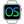HelpWLanguageWLanguage syntaxData typesAdvanced typesNumericPresentationExampleAssigning a numeric variableThousand separatorOperations that can be performed with a numeric typeOperation involving a numeric typeLimitCurrency/Numeric comparisonSee alsoAlias typeBufferCharacter stringsConverting C typesCurrenciesDateDateTimeDifferent types of dataDurationJSONNumericOperations available for the dates, times and durations, ...RealsRectangleThe different types of integersTimeUUIDVariantYAML
 Assigning a numeric variableThousand separatorOperations that can be performed with a numeric typeOperation involving a numeric typeLimitCurrency/Numeric comparison WINDEVWEBDEVWINDEV MobileOthersNumeric (Type of variable) In french: Numérique (Type de variable) The numeric type is used to contain integer or decimal numbers by specifying if necessary:the number of digits in the integer part.the number of digits in the integer part and in the decimal part.A numeric variable can be used:to declare a simple variable.as element of array.as element of composite variable.as element of structure.as element of class.A Variant variable can contain a numeric.Example Counter is numericCounter is numeric (*)Counter is numeric (5,7) num1 is numeric (2,15)num2 is numeric (2,15)num1 = 0n10.000000000000033num2 = 0n10.000000000000055Info(num1+num2) Syntax Declaring a numeric type (default number of digits: 32 digits for the integer part and 6 digits for the decimal part) is numeric, are numeric : Name of the variable to declare. This variable will be a numeric variable with 32 digits for the integer part and 6 digits for the decimal part. Declaring a numeric type (automatic number of digits) is numeric (*), are numeric (*) : Name of the variable to declare. (*) means that the format of the variable is automatically adapted to the value. Declaring a numeric type while specifying the integer part is numeric (), are numeric () : Name of the variable to declare. : Number of digits in the integer part. A numeric can contain up to 38 significant digits. Declaring a numeric type while specifying the integer part and the decimal part is numeric (, ), are numeric (, ) : Name of the variable to declare. : Number of digits in the integer part. A numeric can contain up to 38 significant digits. : Number of digits in the decimal part. A numeric can contain up to 38 significant digits.Remarks Assigning a numeric variableWhen assigning a value (value entered directly, given by a function, etc.) to a numeric value, this value is automatically converted into real by the compiler.For example: num1 is numericnum1 = 1234567890123456789123456789Trace(num1) // num1 does not contain the correct value num2 is numericnum2 = 1234567890123456789.0Trace(num2) // num2 does not contain the correct value To force the assignment of a numeric type, use 0n before the value. For example: num1 is numericnum1 = 0n1234567890123456789123456789Trace(num1) // num1 contains the correct value num2 is numericnum2 = 0n1234567890123456789.0Trace(num2) // num2 contains the correct value Thousand separatorYou can use spaces and underscore characters to separate thousands in numbers. For example: num1 is numericnum1 = 123 456.478num2 is numericnum2 = 0n 47 568 014.478num3 is numericnum3 = 7_014.478 Operations that can be performed with a numeric typeThe following operations can be performed with numeric variables:Comparison operations: ability to use the =, <>, <, <=, >, >= operatorsArithmetic operations: Addition (+), negation (-), subtraction (-), multiplication (-), division (/), modulus.Combined operations: ++, --,+=, -=Operation involving a numeric typeDuring an operation that involves a numeric variable, all the calculations are performed in the format of the numeric values handled.For example, during an operation between a numeric and a real, the real is converted into numeric to perform the operation.LimitA numeric type can have 38 significant digits.The "highest" cases ((0,38) or (1,37) for example) can produce calculations errors caused by overflows.The "lowest" cases ((38,0) or (37,1) for example) can produce calculation errors caused by loss of decimal values.Numbers (real, integer, currency, numeric) cannot exceed 14 significant digits in PHP. If they exceed this limit, they are rounded to 14 digits.Currency/Numeric comparisonThe Currency type is faster for the calculations that do not require a precision greater than 24 significant digits (up to 17 digits for the integer part and up to 6 digits for the decimal part). See also Minimum version required Version 9 This page is also available for…